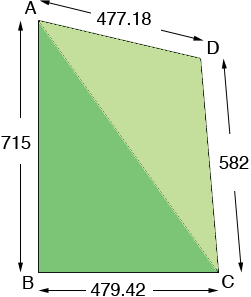SEARCH HOMEMath Central Quandaries & Queriesour land is four sided, but not the same length. We are doing a property line revision and the new measurements are, 479.46 by 715 by477.81 by 582. what will this give me in acreage.Steve,

Unfortunately there isn't enough information here to find the area of your lot. Besides the lengths of the sides I need some information on the shape of the lot. If you can tell me the angle at one of the corners or the length of one of the diagonals then I can find the area.

Harley

Steve wrote back.

715 length by 479.42 with (90degree angle), by 582 length by 477.18 .

Using your description I drew a rough diagram (not to scale). You didn't give me units so I am going to assume they are feet. I labeled the corners so that I can refer to them.The angle at B is a right angle so I can find the area of the triangle AC using the fact that the area of a triangle is half the base times the height. Thus I get

area of triangle ABC =  479.42/2715 = 171392.65 square feet.

Again since triangle ABC is a right triangle I can use Pythagoras theorem to find the length of AC.

|AC|2 = 7152 + 479.422 = 741068.54 and hence |AC| = 860.85 feet.

Now that I have the lengths of all three sides of triangle ACD I can find its area using Heron's formula. I got

area of triangle ACD = 131817.21 square feet.

Thus the area of your lot is

171392.65 + 131817.21 = 303209.86 square feet.

There are 43560 square feet in an acre so the area of your lot is

303209.86/43560 = 6.96 acres.

HarleyMath Central is supported by the University of Regina and The Pacific Institute for the Mathematical Sciences.